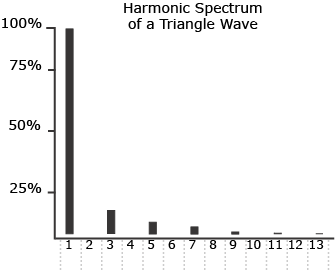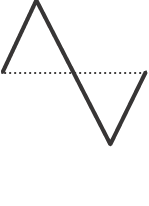## 13. Triangle Waves

The triangle wave is the second common waveform examined in Electronic Music Interactive, and it has the following characteristics:

 Frequency Components Odd Numbered Harmonics Relative Amplitudes of Harmonics 1/Harmonic Number Squared Phase Every Other Harmonic is 180 degrees Out of Phase

The ratio 1/harmonic number squared means that the first harmonic has an amplitude of 1/1, or 1; that the third harmonic will have an amplitude of 1/9 (one ninth the strength of the fundamental); the fifth harmonic will have an amplitude of 1/25 (one twenty-fifth the strength of the fundamental), and so on.Resulting Waveform

Example 13-1: The building of a triangle wave, a common waveform.